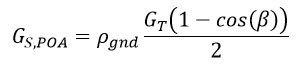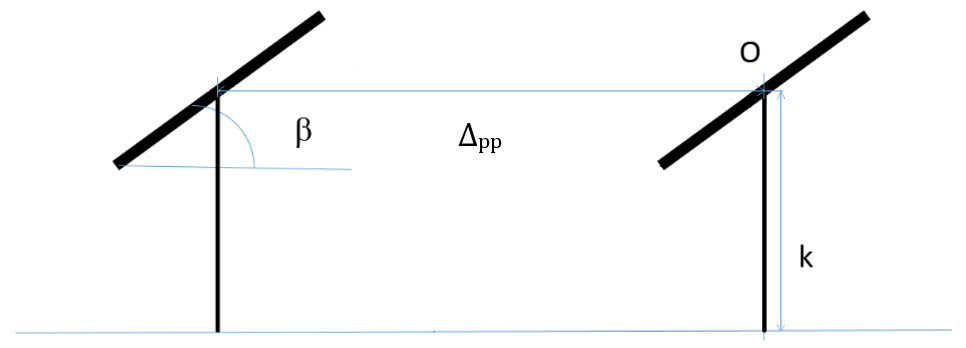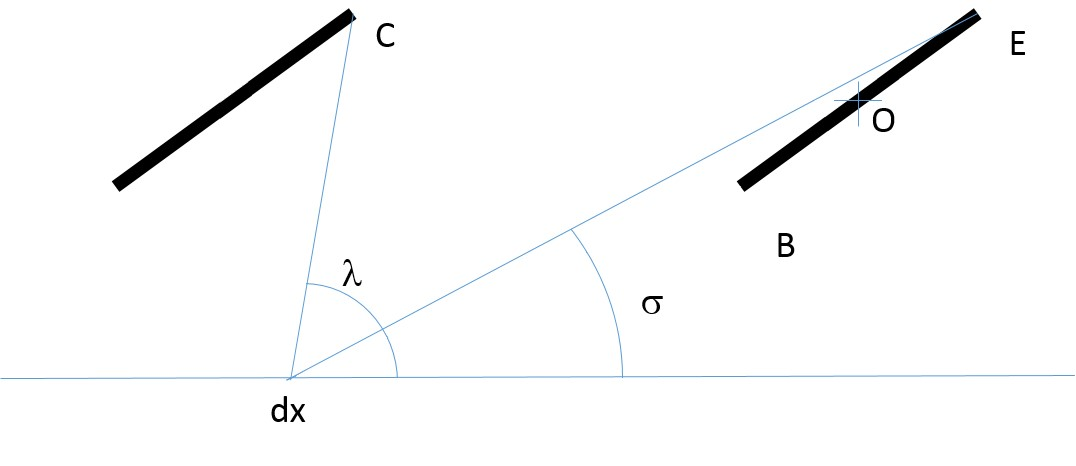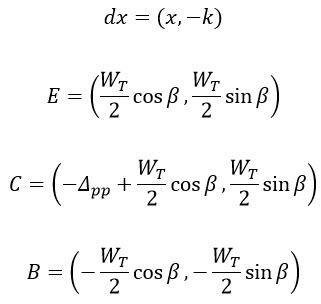This algorithm calculates the effective irradiance from the ground to the module corrected for incidence angle losses for a uniform array (tracker or fixed tilt).

## Algorithm

### Single Array

The irradiance from the ground onto a surface tilted with angle β, where GS,POA is the irradiance on the surface, is:When modeling the ground reflected irradiance on a utility scale solar array, the above equation is insufficient because it does not account for the IAM response of the module surface when receiving ground reflected irradiance. Also, it does not account for row-to-row “ground shading”. The subsequent sections adapt the above equation to account for IAM and ground shading effects.

### Regular Array### Figure 28. Geometry for diffuse ground shading calculation

Consider a two table cross section of a large array as shown in Figure 28. The total irradiance onto the segment between two tables is the sum of a diffuse component and a direct component. The diffuse component is found by calculating the sky view factor between the arrays. The direct component is found using the sun and array angles.  Rewriting the equation for the ground-reflected irradiance for a single plate to include both the diffuse and direct terms results in the following:Where:
Gs,POA-1D  is the irradiance reflected from the ground to the table surface.
GD,1D is the diffuse irradiance on the segment.
GB,1D  is the beam irradiance on the segment.

### GD, 1D, Diffuse

The total diffuse irradiance on a module in a regular array can be approximated by integrating the product of sky-view factor and IAM factor over a reasonable range in front of the module.We assume that the reasonable range is from a point on the row in front of the module (-Δpp where Δpp is row-to-row spacing) to the bottom field of view of the module (the closest point on the ground visible to the module), denoted by P, where P = -k cot β.### Figure 29. Angles and segments used to integrate the ground-view seen in a regular, repeating array.### Figure 30. Additional angles and segments used in the geometric calculations.

In the figures above, point O is the origin. The coordinate system assumes that right and up are positive directions. Thus all values of x, are negative. Although not shown in the figures, WT is the table width (the distance between points E and B).  Below are the calculations of the geometry of the system:

The coordinates are:And the angles are:If λ(x) < 0 then:The sky-view factor is then:The fractional sky-view factor is then:For simplicity, we are using the ASHRAE IAM model:Where the incidence angle,  is:### GB,1D, Direct

In this approximation, only the direct beam hitting the ground in front of the module is considered. The direct beam is a step function dependent on the sun angle with respect to the array. Consequently, the limits of integration are a function of solar and array position.